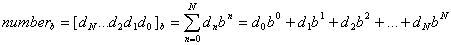# Numeral systems

## Numeral systemb - numeral system base

dn - the n-th digit

n - can start from negative number if the number has a fraction part.

N+1 - the number of digits

## Binary Numeral System - Base-2

Binary numbers uses only 0 and 1 digits.

B denotes binary prefix.

#### Examples:

101012 = 10101B = 1×24+0×23+1×22+0×21+1×20 = 16+4+1= 21

101112 = 10111B = 1×24+0×23+1×22+1×21+1×20 = 16+4+2+1= 23

1000112 = 100011B = 1×25+0×24+0×23+0×22+1×21+1×20 =32+2+1= 35

## Octal Numeral System - Base-8

Octal numbers uses digits from 0..7.

#### Examples:

278 = 2×81+7×80 = 16+7 = 23

308 = 3×81+0×80 = 24

43078 = 4×83+3×82+0×81+7×80= 2247

## Decimal Numeral System - Base-10

Decimal numbers uses digits from 0..9.

These are the regular numbers that we use.

#### Example:

253810 = 2×103+5×102+3×101+8×100

## Hexadecimal Numeral System - Base-16

Hex numbers uses digits from 0..9 and A..F.

H denotes hex prefix.

#### Examples:

2816 = 28H = 2×161+8×160 = 40

2F16 = 2FH = 2×161+15×160 = 47

BC1216 = BC12H = 11×163+12×162+1×161+2×160= 48146

## Numeral systems conversion table

Decimal

Base-10

Binary

Base-2

Octal

Base-8

Base-16

0 0 0 0
1 1 1 1
2 10 2 2
3 11 3 3
4 100 4 4
5 101 5 5
6 110 6 6
7 111 7 7
8 1000 10 8
9 1001 11 9
10 1010 12 A
11 1011 13 B
12 1100 14 C
13 1101 15 D
14 1110 16 E
15 1111 17 F
16 10000 20 10
17 10001 21 11
18 10010 22 12
19 10011 23 13
20 10100 24 14
21 10101 25 15
22 10110 26 16
23 10111 27 17
24 11000 30 18
25 11001 31 19
26 11010 32 1A
27 11011 33 1B
28 11100 34 1C
29 11101 35 1D
30 11110 36 1E
31 11111 37 1F
32 100000 40 20

Currently, we have around 5610 calculators, conversion tables and usefull online tools and software features for students, teaching and teachers, designers and simply for everyone.